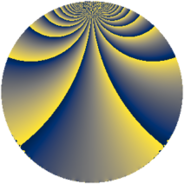Properties

 Label 342.3.yLevel $342$ Weight $3$ Character orbit 342.y Rep. character $\chi_{342}(5,\cdot)$ Character field $\Q(\zeta_{18})$ Dimension $240$ Newform subspaces $1$ Sturm bound $180$ Trace bound $0$

Related objects

Defining parameters

 Level: $$N$$ $$=$$ $$342 = 2 \cdot 3^{2} \cdot 19$$ Weight: $$k$$ $$=$$ $$3$$ Character orbit: $$[\chi]$$ $$=$$ 342.y (of order $$18$$ and degree $$6$$) Character conductor: $$\operatorname{cond}(\chi)$$ $$=$$ $$171$$ Character field: $$\Q(\zeta_{18})$$ Newform subspaces: $$1$$ Sturm bound: $$180$$ Trace bound: $$0$$

Dimensions

The following table gives the dimensions of various subspaces of $$M_{3}(342, [\chi])$$.

Total New Old
Modular forms 744 240 504
Cusp forms 696 240 456
Eisenstein series 48 0 48

Trace form

 $$240 q - 12 q^{3} + 24 q^{6} - 12 q^{9} + O(q^{10})$$ $$240 q - 12 q^{3} + 24 q^{6} - 12 q^{9} - 30 q^{13} + 60 q^{15} + 162 q^{17} + 48 q^{18} + 42 q^{19} - 36 q^{22} - 216 q^{23} + 24 q^{24} + 30 q^{27} - 12 q^{28} - 192 q^{33} + 24 q^{36} + 60 q^{39} - 48 q^{43} + 252 q^{45} + 24 q^{48} - 840 q^{49} + 66 q^{51} - 120 q^{52} - 144 q^{54} + 204 q^{57} - 270 q^{59} - 12 q^{60} + 42 q^{61} - 240 q^{63} + 960 q^{64} - 24 q^{66} + 168 q^{67} - 108 q^{68} - 468 q^{69} - 48 q^{72} + 258 q^{73} + 744 q^{78} + 204 q^{79} - 1212 q^{81} - 144 q^{82} - 216 q^{83} + 180 q^{84} + 708 q^{87} + 648 q^{89} - 24 q^{90} - 192 q^{91} - 480 q^{93} + 648 q^{95} + 180 q^{97} - 648 q^{98} + 1266 q^{99} + O(q^{100})$$

Decomposition of $$S_{3}^{\mathrm{new}}(342, [\chi])$$ into newform subspaces

Label Dim. $$A$$ Field CM Traces $q$-expansion
$a_{2}$ $a_{3}$ $a_{5}$ $a_{7}$
342.3.y.a $240$ $9.319$ None $$0$$ $$-12$$ $$0$$ $$0$$

Decomposition of $$S_{3}^{\mathrm{old}}(342, [\chi])$$ into lower level spaces

$$S_{3}^{\mathrm{old}}(342, [\chi]) \cong$$ $$S_{3}^{\mathrm{new}}(171, [\chi])$$$$^{\oplus 2}$$